Theory and Modern Applications

# Rofe-Beketov formula for symplectic systems

## Abstract

We establish the Rofe-Beketov formula for symplectic systems on time scales. This result generalizes the well-known d’Alembert formula (or the Reduction of Order Theorem) and the Rofe-Beketov formula published for the second order Sturm-Liouville equations on time scales. Moreover, this result is new even in the discrete time case.

MSC:34N05, 26E70, 39A12.

## 1 Introduction

In this article, we solve the open problem presented in , Remark 1(iv)] concerning the Rofe-Beketov formula for symplectic systems on time scales (see Theorem 3), i.e., for the first order dynamic system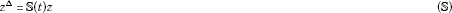on a time scale $\mathbb{T}$. We unify the Rofe-Beketov formulas published recently in the literature for the second order Sturm-Liouville differential, difference, and dynamic equations and also for the linear Hamiltonian differential systems and we generalize them by establishing its dynamic counterpart for system ($\mathbb{S}$). We point out that this result is new even in the discrete time case (see Remark 4(v)) and, moreover, it can be viewed as an improvement of the corresponding Reduction of Order Theorem, see , Remark 6] and , Theorem 3.32], respectively.

Let us consider the second order Sturm-Liouville differential equation

$-{\left(p\left(t\right){x}^{\prime }\right)}^{\prime }+q\left(t\right)x=0,\phantom{\rule{1em}{0ex}}t\in J,$
(1)

where $p>0$ on J and ${p}^{-1}$, $q\in {\mathrm{L}}_{\mathrm{loc}}^{1}\left(J\right)$. Let x be a solution of (1) without zeros in $\stackrel{˜}{J}\subseteq J$ and ${t}_{0}\in \stackrel{˜}{J}$. Then the second linearly independent solution y of (1) on $\stackrel{˜}{J}$ can be expressed with using the so-called d’Alembert formula (or the Reduction of Order Theorem) as

$y\left(t\right)=x\left(t\right){\int }_{{t}_{0}}^{t}\frac{\mathrm{d}s}{p\left(s\right){x}^{2}\left(s\right)},\phantom{\rule{1em}{0ex}}t\in \stackrel{˜}{J},$
(2)

see, e.g., Theorem 5.56], and these solutions are normalized, i.e.,

$w\left[x,y\right]\left(t\right):=x\left(t\right)p\left(t\right){y}^{\prime }\left(t\right)-p\left(t\right){x}^{\prime }\left(t\right)y\left(t\right)\equiv 1,\phantom{\rule{1em}{0ex}}t\in \stackrel{˜}{J}.$

The Rofe-Beketov formula improves identity (2) and it gives a similar result without the assumption $x\left(t\right)\ne 0$ for $t\in \stackrel{˜}{J}$. More precisely, if x is a nontrivial solution of (1) then the function

$y\left(t\right)=x\left(t\right){\int }_{{t}_{0}}^{t}\frac{\left(q\left(s\right)+\frac{1}{p\left(s\right)}\right)\left({x}^{2}\left(s\right)-{\left(p\left(s\right){x}^{\prime }\left(s\right)\right)}^{2}\right)}{{\left({x}^{2}\left(s\right)+{\left(p\left(s\right){x}^{\prime }\left(s\right)\right)}^{2}\right)}^{2}}\phantom{\rule{0.2em}{0ex}}\mathrm{d}s-\frac{p\left(t\right){x}^{\prime }\left(t\right)}{{x}^{2}\left(t\right)+{\left(p\left(t\right){x}^{\prime }\left(t\right)\right)}^{2}},\phantom{\rule{1em}{0ex}}{t}_{0}\in J,$
(3)

represents the second linearly independent solution of equation (1) on J and it holds $w\left[x,y\right]\equiv 1$. This statement was established in , Lemma 2] and it is a generalization of the original Rofe-Beketov formula presented for equation (1) with $p\left(\cdot \right)\equiv 1$ in , Lemma 2]. An application of identity (3) can be found in the study of the relative oscillation theory and spectral properties of differential operators associated with (1), see [5, 7].

In the year 2005, identity (3) was generalized for the linear Hamiltonian differential system

${x}^{\prime }=A\left(t\right)x+B\left(t\right)u,\phantom{\rule{2em}{0ex}}{u}^{\prime }=C\left(t\right)x-{A}^{\ast }\left(t\right)u,\phantom{\rule{1em}{0ex}}t\in J,$
(H)

where A, B, C are $n×n$ matrix-valued locally integrable complex functions and $B={B}^{\ast }$, $C={C}^{\ast }$, see , Theorem 6.5]. This result is recalled in the following proposition, where we denote the Hermitian component of the matrix A by ReA, i.e., $ReA:=\left(A+{A}^{\ast }\right)/2$, see e.g., pp.268-269] or , Facts 3.7.27-3.7.29].

Proposition 1 (Rofe-Beketov formula for (H))

If$\left(\begin{array}{c}X\\ U\end{array}\right)$is a$2n×n$matrix solution of (H) such that${X}^{\ast }U={U}^{\ast }X$and$det\left({X}^{\ast }X+{U}^{\ast }U\right)\ne 0$, then the pair

$\stackrel{˜}{X}=XF-UG,\phantom{\rule{2em}{0ex}}\stackrel{˜}{U}=UF+XG,$

where we put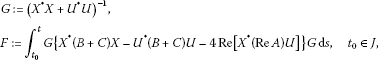forms the second linearly independent$2n×n$matrix-valued solution of (H) satisfying the condition${X}^{\ast }\stackrel{˜}{U}-{U}^{\ast }\stackrel{˜}{X}\equiv I$, i.e., the solutions$\left(\begin{array}{c}X\\ U\end{array}\right)$and$\left(\begin{array}{c}\stackrel{˜}{X}\\ \stackrel{˜}{U}\end{array}\right)$are normalized. Moreover, the conditions${\stackrel{˜}{X}}^{\ast }\stackrel{˜}{U}={\stackrel{˜}{U}}^{\ast }\stackrel{˜}{X}$and$det\left({\stackrel{˜}{X}}^{\ast }\stackrel{˜}{X}+{\stackrel{˜}{U}}^{\ast }\stackrel{˜}{U}\right)\ne 0$hold also true.

The Rofe-Beketov formula was also established for the second order Sturm-Liouville dynamic equation

$-{\left(p\left(t\right){x}^{\mathrm{\Delta }}\right)}^{\mathrm{\Delta }}+q\left(t\right){x}^{\sigma }=0,\phantom{\rule{1em}{0ex}}t\in \mathbb{T},$
(4)

where $\mathbb{T}$ denotes a time scale, see , Theorem 1]. In addition, since this result was new in the discrete time case, i.e., $\mathbb{T}=\mathbb{Z}$, the Rofe-Beketov formula for the second order Sturm-Liouville difference equation

$-\mathrm{\Delta }\left({p}_{k}\mathrm{\Delta }{x}_{k}\right)+{q}_{k}{x}_{k+1}=0$
(5)

was presented in , Remark 1(ii)]. In this article we complete this treatment by proving the Rofe-Beketov formula for symplectic dynamic (and consequently for difference) systems.

## 2 Preliminaries on time scales and symplectic systems

The time scale calculus was originally published by Hilger in his dissertation , see also . It is well known that this theory provides suitable tools for a study of differential and difference (among others) equations under the unified framework. The time scale theory has been developed in the last 20 years intensively and we refer to [13, 14] for the fundamental results achieved in this field.

A time scale$\mathbb{T}$ is any nonempty closed subset of real numbers $\mathbb{R}$. With respect to the standard terminology, the forward jump operator$\sigma :\mathbb{T}\to \mathbb{T}$ and the backward jump operator$\rho :\mathbb{T}\to \mathbb{T}$ are introduced as

$\sigma \left(t\right):=inf\left\{s\in \mathbb{T}|s>t\right\}\phantom{\rule{1em}{0ex}}\text{and}\phantom{\rule{1em}{0ex}}\rho \left(t\right):=sup\left\{s\in \mathbb{T}|s

respectively, and simultaneously we put $inf\mathrm{\varnothing }:=sup\mathbb{T}$ and $sup\mathrm{\varnothing }:=inf\mathbb{T}$. The graininess function$\mu :\mathbb{T}\to \left[0,\mathrm{\infty }\right)$ is defined as $\mu \left(t\right):=\sigma \left(t\right)-t$.

Let $t\in \mathbb{T}$ be a point such that $t>inf\mathbb{T}$. It is said to be left-dense and left-scattered if $\rho \left(t\right)=t$ and $\rho \left(t\right), respectively. On the other hand, a point $t\in \mathbb{T}$ satisfying $t is called right-dense and right-scattered if $\sigma \left(t\right)=t$ and $\sigma \left(t\right)>t$, respectively. In addition, for $a=min\mathbb{T}$ we put $\rho \left(a\right)=a$ and for $b=max\mathbb{T}$ we have $\sigma \left(b\right)=b$. We also use the following notation

For a complex-valued function f and $t\in {\mathbb{T}}^{\kappa }$ we define ${f}^{\mathrm{\Delta }}\left(t\right)$, i.e., the Δ-derivative of f at t, as the number (provided it exists) with the property that for any given $\epsilon >0$ there is a neighborhood $\mathcal{U}$ of t (i.e., $\mathcal{U}=\left(t-\delta ,t+\delta \right)$ for some $\delta >0$) such that

$|{f}^{\sigma }\left(t\right)-f\left(s\right)-{f}^{\mathrm{\Delta }}\left(t\right)\left(\sigma \left(t\right)-s\right)|\le \epsilon |\sigma \left(t\right)-s|,$

where we used the notation ${f}^{\sigma }\left(t\right):=f\left(\sigma \left(t\right)\right)$. We note that ${f}^{\mathrm{\Delta }}\left(b\right)$ is not well defined if $b=max\mathbb{T}$ exists and is left-scattered.

The rule for the differentiation of a product fg has the well-known form

${\left(fg\right)}^{\mathrm{\Delta }}\left(t\right)={f}^{\mathrm{\Delta }}\left(t\right)g\left(t\right)+{f}^{\sigma }\left(t\right){g}^{\mathrm{\Delta }}\left(t\right)={f}^{\mathrm{\Delta }}\left(t\right){g}^{\sigma }\left(t\right)+f\left(t\right){g}^{\mathrm{\Delta }}\left(t\right)$
(6)

and it also holds

${f}^{\sigma }\left(t\right)=f\left(t\right)+\mu \left(t\right){f}^{\mathrm{\Delta }}\left(t\right)$
(7)

whenever ${f}^{\mathrm{\Delta }}\left(t\right)$ exists.

A complex function f is called regressive on $J\subseteq {\mathbb{T}}^{\kappa }$ if

where I denotes an appropriate identity matrix. A function f is called regulated if its right-hand limit $f\left({t}^{+}\right)$ exists (finite) at all right-dense points $t\in \mathbb{T}$ and the left-hand limit $f\left({t}^{-}\right)$ exists (finite) at all left-dense points $t\in \mathbb{T}$. Provided a function f is regulated and it is continuous at each right-dense point $t\in \mathbb{T}$, it is called rd-continuous (we write $f\in {\mathrm{C}}_{\mathrm{rd}}$) on $\mathbb{T}$. A function f is said to be piecewise rd-continuous ($f\in {\mathrm{C}}_{\mathrm{prd}}$) on $\mathbb{T}$ if it is regulated and if f is rd-continuous at all but possibly finitely many right-dense points $t\in \mathbb{T}$. A function f is said to be rd-continuously Δ-differentiable ($f\in {\mathrm{C}}_{\mathrm{rd}}^{\mathrm{1}}$) on $\mathbb{T}$ if ${f}^{\mathrm{\Delta }}$ exists for all $t\in {\mathbb{T}}^{\kappa }$ and ${f}^{\mathrm{\Delta }}\in {\mathrm{C}}_{\mathrm{rd}}$ on ${\mathbb{T}}^{\kappa }$. Finally, a function f is said to be piecewise rd-continuously Δ-differentiable ($f\in {\mathrm{C}}_{\mathrm{prd}}^{\mathrm{1}}$) on ${\left[a,b\right]}_{\mathbb{T}}$ if f is continuous on $\mathbb{T}$ and ${f}^{\mathrm{\Delta }}\left(t\right)$ exists at all except of possibly finitely many points $t\in {\mathbb{T}}^{\kappa }$, and ${f}^{\mathrm{\Delta }}\in {\mathrm{C}}_{\mathrm{prd}}$ on ${\mathbb{T}}^{\kappa }$.

It is also well known that for any rd-continuous function on $\mathbb{T}$ there exists an antiderivative F, i.e., a function satisfying ${F}^{\mathrm{\Delta }}\left(t\right)=f\left(t\right)$ for all $t\in {\mathbb{T}}^{\kappa }$. A time scale integral of a function f over a time scale interval $\left[c,d\right]\cap \mathbb{T}$, where $c,d\in \mathbb{T}$, is defined as

${\int }_{c}^{d}f\left(t\right)\mathrm{\Delta }t:=F\left(d\right)-F\left(c\right)$

for any antiderivative F of f.

In this article, we are interested in the symplectic system on time scaleswhere the $2n×2n$ matrix-valued complex function $\mathbb{S}\left(\cdot \right)$ satisfies

(8)

With using the block notation $Z=\left(\begin{array}{c}X\\ U\end{array}\right)$ and $\mathbb{S}\left(t\right):=\left(\begin{array}{cc}\mathbb{A}\left(t\right)& \mathbb{B}\left(t\right)\\ \mathbb{C}\left(t\right)& \mathbb{D}\left(t\right)\end{array}\right)$, system ($\mathbb{S}$) can be written in the form

${X}^{\mathrm{\Delta }}=\mathbb{A}\left(t\right)X+\mathbb{B}\left(t\right)U,\phantom{\rule{2em}{0ex}}{U}^{\mathrm{\Delta }}=\mathbb{C}\left(t\right)X+\mathbb{D}\left(t\right)U,$

where $X,U\in {\mathrm{C}}_{\mathrm{prd}}^{\mathrm{1}}$ are $n×m$, $1\le m\le n$, matrix-valued complex functions and the coefficients are $n×n$ matrix-valued complex functions such that $\mathbb{A},\mathbb{B},\mathbb{C},\mathbb{D}\in {\mathrm{C}}_{\mathrm{prd}}$ on $\mathbb{T}$. Identity (8) can be also written in this block notation as (we omit the argument t)

$\begin{array}{lcr}{\mathbb{B}}^{\ast }-\mathbb{B}+\mu \left({\mathbb{B}}^{\ast }\mathbb{D}-{\mathbb{D}}^{\ast }\mathbb{B}\right)& =& 0,\\ {\mathbb{C}}^{\ast }-\mathbb{C}+\mu \left({\mathbb{C}}^{\ast }\mathbb{A}-{\mathbb{A}}^{\ast }\mathbb{C}\right)& =& 0,\\ {\mathbb{A}}^{\ast }+\mathbb{D}+\mu \left({\mathbb{A}}^{\ast }\mathbb{D}-{\mathbb{C}}^{\ast }\mathbb{B}\right)& =& 0.\end{array}\right\}$
(9)

Moreover, we note that identity (8) implies the symplecticity of the matrix $\left(I+\mu \mathbb{S}\right)$ on ${\mathbb{T}}^{\kappa }$, i.e., ${\left(I+\mu \mathbb{S}\right)}^{\ast }\mathcal{J}\left(I+\mu \mathbb{S}\right)=\mathcal{J}$. Since every symplectic matrix is invertible, it follows that the matrix-valued function $\mathbb{S}\left(\cdot \right)$ is regressive on ${\mathbb{T}}^{\kappa }$. Hence the existence of a unique solution for any (vector or matrix) initial value problem is a consequence of , Theorem 5.7] or , Theorem 5.8], see also , Remark 1].

The theory of symplectic difference systems was initiated in  as the discrete counterpart of system (H), while the study of system ($\mathbb{S}$) originates in . It is a well-known fact that system ($\mathbb{S}$) includes many special cases, e.g., equations (1), (4) and (5), system (H), discrete symplectic systems, and any even order Sturm-Liouville equation, see .

We associate with system ($\mathbb{S}$) the Wronskian matrix given by

$W\left[Z,\stackrel{˜}{Z}\right]\left(t\right):={X}^{\ast }\left(t\right)\stackrel{˜}{U}\left(t\right)-{U}^{\ast }\left(t\right)\stackrel{˜}{X}\left(t\right)=\left(\begin{array}{c}X\left(t\right)\\ U\left(t\right)\end{array}{\right)}^{\ast }\mathcal{J}\left(\begin{array}{c}\stackrel{˜}{X}\left(t\right)\\ \stackrel{˜}{U}\left(t\right)\end{array}\right),$

where $Z=\left(\begin{array}{c}X\\ U\end{array}\right)$ and $\stackrel{˜}{Z}=\left(\begin{array}{c}\stackrel{˜}{X}\\ \stackrel{˜}{U}\end{array}\right)$ are any $2n×m$, $1\le m\le n$, solutions of ($\mathbb{S}$). It is a direct consequence of ${W}^{\mathrm{\Delta }}\left[Z,\stackrel{˜}{Z}\right]\equiv 0$ that the Wronskian matrix takes a constant value on $\mathbb{T}$.

A solution $Z=\left(\begin{array}{c}X\\ U\end{array}\right)$ of ($\mathbb{S}$) is said to be a conjoined solution if $W\left[Z,Z\right]\equiv 0$, i.e., ${X}^{\ast }\left(t\right)U\left(t\right)$ is a Hermitian matrix at one (and hence at any) $t\in \mathbb{T}$. Two solutions $Z,\stackrel{˜}{Z}$ are called normalized if $W\left[Z,\stackrel{˜}{Z}\right]\equiv I$. A solution $Z=\left(\begin{array}{c}X\\ U\end{array}\right)$ is said to be a basis if $rankZ=n$ on $\mathbb{T}$. It was shown in , Proposition 2.2] that the value of $rankZ\left(t\right)$ is also constant on $\mathbb{T}$.

## 3 Main result

The Reduction of Order Theorem for system ($\mathbb{S}$) was published in , Remark 6] and it is recalled in the following proposition.

Proposition 2 (Reduction of Order Theorem for ($\mathbb{S}$))

Let$Z=\left(\begin{array}{c}X\\ U\end{array}\right)$be a conjoined basis of ($\mathbb{S}$) such that X is invertible on the time scale interval$I:=\left[a,b\right]\cap \mathbb{T}$, $a,b\in \mathbb{T}$. Then$\stackrel{˜}{Z}=\left(\begin{array}{c}\stackrel{˜}{X}\\ \stackrel{˜}{U}\end{array}\right)$, where

$\begin{array}{l}\stackrel{˜}{X}:=X\left(t\right){\int }_{a}^{t}\left\{{\left({X}^{\sigma }\right)}^{-1}\mathbb{B}{X}^{\ast -1}\right\}\left(s\right)\mathrm{\Delta }s,\\ \stackrel{˜}{U}:=U\left(t\right){\int }_{a}^{t}\left\{{\left({X}^{\sigma }\right)}^{-1}\mathbb{B}{X}^{\ast -1}\right\}\left(s\right)\mathrm{\Delta }s+{X}^{\ast -1}\left(t\right),\end{array}\right\}\phantom{\rule{1em}{0ex}}t\in \mathbb{T},$

is also a conjoined basis of ($\mathbb{S}$). Moreover, Z and$\stackrel{˜}{Z}$are linearly independent (even normalized), i.e., they form a basis of the solution space for ($\mathbb{S}$).

Now, we improve this proposition in the main result by dropping the invertibility of X, i.e., we state and prove the Rofe-Beketov formula for system ($\mathbb{S}$).

Theorem 3 (Rofe-Beketov formula for ($\mathbb{S}$))

Let$Z=\left(\begin{array}{c}X\\ U\end{array}\right)$be a conjoined basis of ($\mathbb{S}$). Then the pair$\stackrel{˜}{Z}=\left(\begin{array}{c}\stackrel{˜}{X}\\ \stackrel{˜}{U}\end{array}\right)$given by

$\stackrel{˜}{X}=XF-UG,\phantom{\rule{2em}{0ex}}\stackrel{˜}{U}=XG+UF,$
(10)

where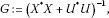(11)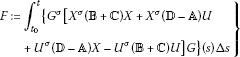(12)

for a fixed${t}_{0}\in \mathbb{T}$chosen without any restriction, solves also system ($\mathbb{S}$). Moreover, $\stackrel{˜}{Z}$is a basis of ($\mathbb{S}$) and it holds$W\left[Z,\stackrel{˜}{Z}\right]\equiv I$ (i.e., they are linearly independent and normalized). In addition, $\stackrel{˜}{Z}$constitutes also a conjoined basis if${F}^{\ast }=F$.

Proof The proof is based on the variation of parameters method. By differentiating identity (10)(i), and from (6), we get

${\stackrel{˜}{X}}^{\mathrm{\Delta }}={X}^{\mathrm{\Delta }}F+{X}^{\sigma }{F}^{\mathrm{\Delta }}-{U}^{\mathrm{\Delta }}G-{U}^{\sigma }{G}^{\mathrm{\Delta }}.$
(13)

Since $Z=\left(\begin{array}{c}X\\ U\end{array}\right)$ solves system ($\mathbb{S}$) and $\stackrel{˜}{Z}=\left(\begin{array}{c}\stackrel{˜}{X}\\ \stackrel{˜}{U}\end{array}\right)$ has to solve the same system, it follows from (13) that

${X}^{\sigma }{F}^{\mathrm{\Delta }}-{U}^{\sigma }{G}^{\mathrm{\Delta }}={\stackrel{˜}{X}}^{\mathrm{\Delta }}-{X}^{\mathrm{\Delta }}F+{U}^{\mathrm{\Delta }}G=\left[\left(\mathbb{B}+\mathbb{C}\right)X+\left(\mathbb{D}-\mathbb{A}\right)U\right]G.$
(14)

Similarly, we obtain

${U}^{\sigma }{F}^{\mathrm{\Delta }}+{X}^{\sigma }{G}^{\mathrm{\Delta }}={\stackrel{˜}{U}}^{\mathrm{\Delta }}-{U}^{\mathrm{\Delta }}F-{X}^{\mathrm{\Delta }}G=-\left[\left(\mathbb{A}-\mathbb{D}\right)X+\left(\mathbb{B}+\mathbb{C}\right)U\right]G.$
(15)

Since Z is a conjoined basis, the multiplication of identity (14) by ${X}^{\sigma }$ from the left and identity (15) by ${U}^{\sigma }$ from the left yields in the sum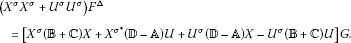(16)

Analogously, multiplying identity (14) by $-{U}^{\sigma }$ from the left and identity (15) by ${X}^{\sigma }$ from the left, we get the dynamic equation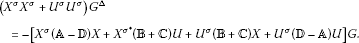Nevertheless, we can get the form of G explicitly. If we multiply identity (10)(i) from the left by $-{U}^{\ast }$ and identity (10)(ii) from the left by ${X}^{\ast }$, we obtain by adding these equations

${X}^{\ast }\stackrel{˜}{U}-{U}^{\ast }\stackrel{˜}{X}=\left({X}^{\ast }U-{U}^{\ast }X\right)F+\left({X}^{\ast }X+{U}^{\ast }U\right)G.$

Since Z is a conjoined basis and it has to be satisfied $W\left[Z,\stackrel{˜}{Z}\right]\equiv I$, it follows

i.e., G is determined by (11). Consequently, identity (16) yields that the matrix-valued function F is given by (12) for a fixed ${t}_{0}\in \mathbb{T}$ chosen without any restriction at the outset of the proof.

Now, from the facts that the value of $rank\stackrel{˜}{Z}$ is constant and the matrix G has a full rank, and since it holds

$\left(\begin{array}{cc}\stackrel{˜}{X}\left({t}_{0}\right)& \stackrel{˜}{U}\left({t}_{0}\right)\end{array}\right)=\left(\begin{array}{cc}-U\left({t}_{0}\right)G\left({t}_{0}\right)& \phantom{-}X\left({t}_{0}\right)G\left({t}_{0}\right)\end{array}\right)=\left(\begin{array}{cc}X\left({t}_{0}\right)& U\left({t}_{0}\right)\end{array}\right)\left(\begin{array}{cc}0& G\left({t}_{0}\right)\\ -G\left({t}_{0}\right)& 0\end{array}\right),$

it follows that the solution $\stackrel{˜}{Z}$ forms a basis of ($\mathbb{S}$), i.e., $rank\stackrel{˜}{Z}=n$ (see also Remark (4)(i)). Moreover, from (10), (11), identity ${X}^{\ast }U={U}^{\ast }X$, and by the direct calculation, we obtain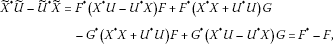i.e., $\stackrel{˜}{Z}$ is also a conjoined solution if ${F}^{\ast }=F$ on $\mathbb{T}$, and the proof is complete. □

Remark 4

1. (i)

The fact that $\stackrel{˜}{Z}$ represents a basis of ($\mathbb{S}$), follows also from the calculation

${\stackrel{˜}{X}}^{\ast }\stackrel{˜}{X}+{\stackrel{˜}{U}}^{\ast }\stackrel{˜}{U}={F}^{\ast }{G}^{-1}F+{G}^{\ast }$

since the condition $rank\stackrel{˜}{Z}=n$ is equivalent with $det\left({\stackrel{˜}{X}}^{\ast }\stackrel{˜}{X}+{\stackrel{˜}{U}}^{\ast }\stackrel{˜}{U}\right)\ne 0$. This condition is obviously satisfied at $t={t}_{0}$ and hence it holds true for any $t\in \mathbb{T}$.

1. (ii)

With using the block identities in (9) and identity (7), the function F can be also given in the form

$\begin{array}{rcl}F& =& {\int }_{{t}_{0}}^{t}\left\{{G}^{\sigma }\left[{X}^{\ast }\left({\mathbb{C}}^{\ast }+\mathbb{B}+\mu \left({\mathbb{A}}^{\ast }\mathbb{B}+{\mathbb{C}}^{\ast }\mathbb{D}\right)\right)X\\ -{X}^{\ast }\left({\mathbb{A}}^{\ast }+\mathbb{A}+\mu \left({\mathbb{A}}^{\ast }\mathbb{A}+{\mathbb{C}}^{\ast }\mathbb{C}\right)\right)U\\ +{U}^{\ast }\left({\mathbb{D}}^{\ast }+\mathbb{D}+\mu \left({\mathbb{B}}^{\ast }\mathbb{B}+{\mathbb{D}}^{\ast }\mathbb{D}\right)\right)X\\ -{U}^{\ast }\left({\mathbb{B}}^{\ast }+\mathbb{C}+\mu \left({\mathbb{B}}^{\ast }\mathbb{A}+{\mathbb{D}}^{\ast }\mathbb{C}\right)\right)U\right]G\right\}\left(s\right)\mathrm{\Delta }s.\end{array}$

On the other hand, with using identity (7) for system ($\mathbb{S}$), i.e., ${Z}^{\sigma }=\left(I+\mu \mathbb{S}\right)Z$, and without the block notation (i.e., only with Z, $\stackrel{˜}{Z}$, and $\mathbb{S}$) the coefficient matrices in (11)-(12) can be written as $G={\left({Z}^{\ast }Z\right)}^{-1}$ and

$\begin{array}{l}F=-{\int }_{{t}_{0}}^{t}\left\{{\left[{Z}^{\ast }\left(I+\mu \left(\mathbb{S}+{\mathbb{S}}^{\ast }+\mu {\mathbb{S}}^{\ast }\mathbb{S}\right)\right)Z\right]}^{-1}\\ \phantom{F=}×\left[{Z}^{\ast }\left(\mathbb{S}+{\mathbb{S}}^{\ast }+\mu {\mathbb{S}}^{\ast }\mathbb{S}\right)\mathcal{J}Z\right]{\left({Z}^{\ast }Z\right)}^{-1}\right\}\left(s\right)\mathrm{\Delta }s.\end{array}\right\}$
(17)
1. (iii)

It follows from identities (17) and (8) that it is satisfied ${F}^{\ast }\left(t\right)=F\left(t\right)$ for a point $t\in \mathbb{T}$ and, consequently, the pair $\stackrel{˜}{Z}$ forms a conjoined basis if it holds $\mu \left(t\right)=0$. Especially if $\mathbb{T}=\mathbb{R}$, we have $\sigma \left(t\right)=t$, $\mu \left(t\right)=0$, and ${f}^{\mathrm{\Delta }}\left(t\right)={f}^{\prime }\left(t\right)$. In this setting, system ($\mathbb{S}$) has the form of (H) and the statement of Theorem 3 corresponds to Proposition 1, i.e., , Theorem 6.5].

2. (iv)

Equation (4), where $1/p,q\in {\mathrm{C}}_{\mathrm{prd}}$, can be written as the symplectic dynamic system

$\left(\begin{array}{c}x\\ p{x}^{\mathrm{\Delta }}\end{array}{\right)}^{\mathrm{\Delta }}=\left(\begin{array}{cc}0& 1/p\\ q& \mu q/p\end{array}\right)\left(\begin{array}{c}x\\ p{x}^{\mathrm{\Delta }}\end{array}\right).$

Theorem 3 yields for a nontrivial solution x of (4) that the second linearly independent solution y such that $p\left(x{y}^{\mathrm{\Delta }}-{x}^{\mathrm{\Delta }}y\right)\equiv 1$, can be expressed as

$y\left(t\right)=f\left(t\right)x\left(t\right)-g\left(t\right)p\left(t\right){x}^{\mathrm{\Delta }}\left(t\right),$

where we put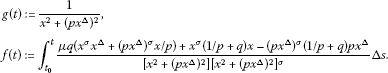This result corresponds to , Theorem 1] by a direct calculation using identity (7) and reduces to , Lemma 2] in the special case $\mathbb{T}=\mathbb{R}$.

1. (v)

As mentioned in the introduction, Theorem 3 is new even in the discrete time case, i.e., for $\mathbb{T}=\mathbb{Z}$. In this case, system ($\mathbb{S}$) can be written as the discrete symplectic system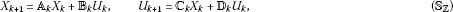where we put ${\mathbb{A}}_{k}:=\mathbb{A}\left(k\right)+I$, ${\mathbb{B}}_{k}:=\mathbb{B}\left(k\right)$, ${\mathbb{C}}_{k}:=\mathbb{C}\left(k\right)$, and ${\mathbb{D}}_{k}:=\mathbb{D}\left(k\right)+I$. Hence, if $Z=\left(\begin{array}{c}X\\ U\end{array}\right)$ is a conjoined basis of (${\mathbb{S}}_{\mathbb{Z}}$), then the pair $\stackrel{˜}{Z}=\left(\begin{array}{c}\stackrel{˜}{X}\\ \stackrel{˜}{U}\end{array}\right)$ given by (10), where

${G}_{k}:={\left({X}_{k}^{\ast }{X}_{k}+{U}_{k}^{\ast }{U}_{k}\right)}^{-1}={\left({Z}_{k}^{\ast }{Z}_{k}\right)}^{-1}$

and

$\begin{array}{rcl}{F}_{k}& :=& \sum _{i={k}_{0}}^{k-1}\left\{{G}_{i+1}\left[{X}_{i+1}^{\ast }\left({\mathbb{B}}_{i}+{\mathbb{C}}_{i}\right){X}_{i}+{X}_{i+1}^{\ast }\left({\mathbb{D}}_{i}-{\mathbb{A}}_{i}\right){U}_{i}\\ +{U}_{i+1}^{\ast }\left({\mathbb{D}}_{i}-{\mathbb{A}}_{i}\right){X}_{i}-{U}_{i+1}^{\ast }\left({\mathbb{B}}_{i}+{\mathbb{C}}_{i}\right){U}_{i}\right]{G}_{i}\right\}\\ =& -\sum _{i={k}_{0}}^{k-1}\left\{{\left({Z}_{i}^{\ast }{\mathbb{S}}_{i}^{\ast }{\mathbb{S}}_{i}{Z}_{i}\right)}^{-1}\left[{Z}_{i}^{\ast }\left({\mathbb{S}}_{i}^{\ast }{\mathbb{S}}_{i}-I\right)\mathcal{J}{Z}_{i}\right]{\left({Z}_{i}^{\ast }{Z}_{i}\right)}^{-1}\right\}\end{array}$

with ${\mathbb{S}}_{k}=\left(\begin{array}{cc}{\mathbb{A}}_{k}& {\mathbb{B}}_{k}\\ {\mathbb{C}}_{k}& {\mathbb{D}}_{k}\end{array}\right)$, represents a solution of (${\mathbb{S}}_{\mathbb{Z}}$) such that $W\left[Z,\stackrel{˜}{Z}\right]=I$, compare with , Theorem 3.32].

## References

1. Došlý O: Rofe-Beketov’s formula on time scales. Comput. Math. Appl. 2010, 60(8):2382–2386. doi:10.1016/j.camwa.2010.08.033 10.1016/j.camwa.2010.08.033

2. Došlý O, Hilscher R: Disconjugacy, transformations and quadratic functionals for symplectic dynamic systems on time scales. J. Differ. Equ. Appl. 2001, 7(2):265–295. doi:10.1080/10236190108808273 10.1080/10236190108808273

3. Ahlbrandt CD, Peterson AC: Discrete Hamiltonian Systems: Difference Equations, Continued Fractions, and Riccati Equations. Kluwer Academic, Dordrecht; 1996.

4. Kelley WG, Peterson AC: The Theory of Differential Equations. Classical and Qualitative. 2nd edition. Springer, New York; 2010.

5. Schmidt KM: Critical coupling constants and eigenvalue asymptotics of perturbed periodic Sturm-Liouville operators. Commun. Math. Phys. 2000, 211(2):465–485. doi:10.1007/s002200050822 10.1007/s002200050822

6. Rofe-Beketov FS: Constants of Kneser type and effective masses for zone potentials. Dokl. Akad. Nauk SSSR 1984, 276(2):356–359.

7. Krüger H, Teschl G: Effective Prüfer angles and relative oscillation criteria. J. Differ. Equ. 2008, 245(12):3823–3848. doi:10.1016/j.jde.2008.06.004 10.1016/j.jde.2008.06.004

8. Rofe-Beketov FS, Kholkin AM: Spectral Analysis of Differential Operators. Interplay between Spectral and Oscillatory Properties. World Scientific, Hackensack; 2005.

9. Gantmacher FR: The Theory of Matrices. AMS Chelsea Publishing, Providence; 1998. vols. I and II

10. Bernstein DS: Matrix Mathematics. Theory, Facts, and Formulas. 2nd edition. Princeton University Press, Princeton; 2009.

11. Hilger, S: Ein Maßkettenkalkül mit Anwendung auf Zentrumsmannigfaltigkeiten. PhD thesis, University of Würzburg (1988)

12. Hilger S: Analysis on measure chains - a unified approach to continuous and discrete calculus. Results Math. 1990, 18: 18–56.

13. Bohner M, Peterson AC: Dynamic Equations on Time Scales. An Introduction with Applications. Birkhäuser, Boston; 2001.

14. Bohner M, Peterson AC (Eds): Advances in Dynamic Equations on Time Scales. Birkhäuser, Boston; 2003.

15. Ahlbrandt CD: Equivalence of discrete Euler equations and discrete Hamiltonian systems. J. Math. Anal. Appl. 1993, 180(2):498–517. doi:10.1006/jmaa.1993.1413 10.1006/jmaa.1993.1413

16. Zemánek P: A note on the equivalence between even-order Sturm-Liouville equations and symplectic systems on time scales. Appl. Math. Lett. 2012. doi:10.1016/j.aml.2012.04.009

17. Hilscher R, Zemánek P: Trigonometric and hyperbolic systems on time scales. Dyn. Syst. Appl. 2009, 18(3–4):483–506.

## Acknowledgements

The research was supported by the Czech Science Foundation under grant P201/10/1032 and also by the Operational Programme “Education for Competitiveness” under the project CZ.1.07/2.3.00/30.0009. The author wishes to thank the anonymous referees for the detailed reading of the manuscript and for their comments which helped to improve the presentation of the results.

## Author information

Authors

### Corresponding author

Correspondence to Petr Zemánek.

### Competing interests

The author declares that he has no competing interests.

## Rights and permissions

Reprints and Permissions

Zemánek, P. Rofe-Beketov formula for symplectic systems. Adv Differ Equ 2012, 104 (2012). https://doi.org/10.1186/1687-1847-2012-104

• Accepted:

• Published:

• DOI: https://doi.org/10.1186/1687-1847-2012-104

### Keywords

• Rofe-Beketov formula
• symplectic system
• time scale
• Reduction of Order Theorem